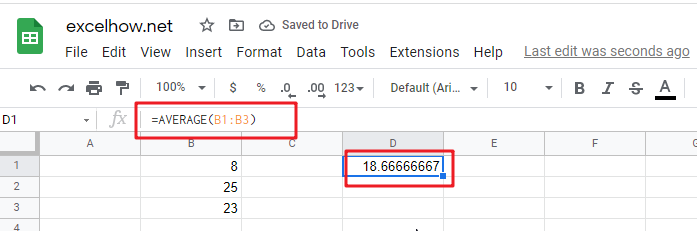# ExcelHow

This post will guide you how to use Google Sheets AVERAGE function with syntax and examples in Google Spreadsheets.

## Description

The Google Sheets AVERAGE function returns the average of the numbers that you provided.

The AVERAGE function is a build-in function in Google Sheets and it is categorized as a Statistical Function.

## Syntax

The syntax of the AVERAGE function is as below:

`= AVERAGE (number1,[number2],…)`

Where the AVERAGE function arguments are:

• `Number1 `-This is a required argument.  the first numeric values
• `Number2 `– This is an optional argument.

## Google Sheets AVERAGE Function Example

The below examples will show you how to use Google Sheets AVERAGE Function to return the average of the numbers provided.

#1 To get the average value of the numbers in cell range B1:B3 Cell, just using the following Google Sheets formula:

`=AVERAGE(B1:B3)`